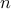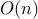# Fast Algorithms for Resource Allocation in Wireless Cellular Networks

R. Madan, S. Boyd, and S. Lall

IEEE/ACM Transactions on Networking, 18(3):973-984, June 2010.

We consider a scheduled orthogonal frequency division multiplexed (OFDM) wireless cellular network where the channels from the base-station to themobile users undergo flat fading. Spectral resources are to be divided among the users in order to maximize total user utility. We show that this problem can be cast as a nonlinear convex optimization problem, and describe analgorithm to solve it. Computational experiments show that the algorithm typically converges in around 25 iterations, where each iteration has a cost that is, with a modest constant. When the algorithm starts from an initial resource allocation that is close to optimal, convergence typically takes even fewer iterations. Thus, the algorithm can efficiently track the optimal resource allocation as the channel conditions change due to fading. We also show how our techniques can be extended to solve resource allocation problems that arise in wideband networks with frequency selective fading and when the utility of a user is also a function of the resource allocations in the past.